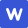|

# 文科数学 热门试卷

X 查看更多试卷

1、单选题
1
2
3
4
5
6
7
8
9
10
11
12
2、填空题
13
14
15
16
3、简答题
17
18
19
20
21
22
• 真题试卷
• 模拟试卷
• 预测试卷

1

|

4.已知，若A，B，C三点共线，则实数k的值为(  )

A4

B﹣4

C

D

C

【分值】5

### 易错点

1

|

1.设集合A={x|﹣2≤x≤3}，B={x|x+1＞0}，则集合A∩B等于(  )

A{x|﹣2≤x≤﹣1}

B{x|﹣2≤x＜﹣1}

C{x|﹣1＜x≤3}

D{x|1＜x≤3}

C

### 解析

∵集合A={x|-2≤x≤3}，

B={x|x+1＞0}={x|x＞-1}，∴A∩B={x|-1＜x≤3}．故选C

### 易错点

1

|

2.复数满足，则在复平面内，复数对应的点位于(  )

A第一象限

B第二象限

C第三象限

D第四象限

C

### 解析

，对应的点为(-1，-3)．故选C．

### 易错点

1

|

3.春节前，某市一过江大桥上挂了两串彩灯，这两串彩灯的第一次闪亮相互独立，且都在通电后的6秒内任一时刻等可能发生，然后每串彩灯以6秒内间隔闪亮，那么这两串彩灯同时通电后，它们第一次闪亮的时刻相差不超过3秒的概率是(  )

A

B

C

D

B

,

.

### 易错点

1

|

5.设等差数列{an}的前n项和为Sn，若a2+a4+a9=24，则S9=(  )

A36

B72

C144

D70

B

### 解析

∵{an}为等差数列，设首项为a1，公差为d，

∴a2+a4+a9=3a1+12d=24，即a1+4d=8，

∵S9=9a1+

d=9×(a1+4d)=9×8=72，

s9=a1+a2+a3+a4+a5+a6+a7+a8+a9=9a5=72

### 易错点

1

|

6.已知函数f(x)=3cos(﹣ωx)(ω＞0)，函数f(x)相邻两个零点之间的绝对值为，则下列为函数f(x)的单调递减区间的是(  )

A[0，]

B[，π]

C[]

D[]

C

### 易错点

1

|

7.设不等式4x﹣m(4x+2x+1)≥0对于任意的x∈[0，1]恒成立，则实数m的取值范围是(  )

A(﹣∞，]

B[]

C[]

D[，+∞)

A

### 易错点

1

|

8.如图为某几何体的三视图，则该几何体的表面积为(  )

A10+

B10+

C6+2+

D6++

C

### 易错点

1

|

9.已知双曲线C：=1(a＞0，b＞0)的左、右焦点分别为F1，F2，过F2作平行于C的渐近线的直线交C于点P．若PF1⊥PF2，则C的离心率为(  )

A

B

C2

D

D

### 易错点

1

|

12.对于三次函数f(x)=ax3+bx2+cx+d(a≠0)，给出定义：设f′(x)是函数y=f(x)的导数，f″(x)是f′(x)的导数，若方程f″(x)=0有实数解x0，则称点(x0，f(x0))为函数y=f(x)的“拐点”．某同学经过探究发现：任何一个三次函数都有“拐点”；任何一个三次函数都有对称中心，且“拐点”就是对称中心，设函数g(x)=x3x2+3x﹣，则g()+g()+…+g()=(  )

A2013

B2014

C2015

D2016

B

### 易错点

1

|

10.某程序框图如图所示，若输出的S=120，则判断框内应填入(  )

Ak＞4？

Bk＞5？

Ck＞6？

Dk＞7？

B

### 易错点

1

|

11.已知函数f(x)是定义在R上的奇函数，f(x+2)=f(x)，当x∈(0，1]时，f(x)=1﹣2|x﹣|，则函数g(x)=f(f(x))﹣x在区间内不同的零点个数是(  )

A5

B6

C7

D9

A

### 易错点

1

|

14.已知变量满足约束条件，则的最大值是

9

### 易错点

1

|

16.已知椭圆，过右焦点F且斜率为k(k＞0)的直线与C相交于A、B两点，若=

.

### 解析

＋3 ＝c 2 ，①    ＋3 ＝c 2 ，②

①－9×②，得 (x 1 ＋3x 2 )(x 1 －3x 2 )＋3(y 1 ＋3y 2 )(y 1 －3y 2 )＝－8c 2 ，所以 ×4c(x 1 －3x 2)＝－8c 2 ，

### 易错点

1

|

13.若命题“存在x∈R，使得2x2﹣3ax+9＜0成立”为假命题，则实数a的取值范围是  ___

[﹣2，2]

### 解析

“存在x∈R，使2x2-3ax+9＜0”是假命题，

∴-2≤a≤2

### 易错点

1

|

15.已知m、n是两条不重合的直线，α、β、γ是三个两两不重合的平面，给出下列四个命题，其中所有正确命题的序号是___________．

①若m∥β，n∥β，m、nα，则α∥β .

②若α⊥γ，β⊥γ，α∩β＝m，nγ，则m⊥n .

③若m⊥α，α⊥β，m∥n，则n∥β .

④若n∥α，n∥β，α∩β＝m，那么m∥n .

②④

### 解析

①     若m∥β，n∥β，m、nα，则α∥β；错误

②若α⊥γ，β⊥γ，α∩β＝m，nγ，则m⊥n；成立

③若m⊥α，α⊥β，m∥n，则n∥β或nβ；错误.

④若n∥α，n∥β，α∩β＝m，那么m∥n；成立

### 易错点

1

|

24.求椭圆C的标准方程；

25.若动点B在直线l：y=上，且OA⊥OB，点O到直线AB的距离为d(A，B)，求证：d(A，B)为定值．

=1．

∴椭圆C的标准方程为=1．

△AF1F2的周长为2a+2c

1

### 解析

∵OA⊥OB，∴=0．∴x1=﹣

①若x1≠x0，kAB=，直线AB的方程为：y﹣=(x﹣x1)，

x+(x1﹣x0)y+﹣x1y0=0，

∴d(A，B)=

∴[d(A，B)]2==

∴[d(A，B)]2===1，

∴d(A，B)=1，为定值．

②若x1=x0，设直线OA的方程为：y=kx，则B，A

∴直线AB的方程为：x=±1，点O到直线AB的距离d(A，B)=1．

### 易错点

1

|

17.求数列{an}和{bn}的通项公式；

18.设cn=an•bn，设Tn为{cn}的前n项和，求Tn

an=2n﹣1，bn=3n

### 解析

∵数列{bn}的前n项和Sn满足Sn=(bn﹣1)，

∴b1=S1=，解得b1=3．

∴数列{bn}为等比数列，

∵a2=b1=3，a5=b2=9．

，解得d=2，a1=1．

∴an=2n﹣1．

### 解析

(Ⅱ)cn=an•bn=(2n﹣1)•3n

∴Tn=3+3×32+5×33+…+(2n﹣3)•3n﹣1+(2n﹣1)•3n

3Tn=32+3×33+…+(2n﹣3)•3n+(2n﹣1)•3n+1

∴﹣2Tn=3+2×32+2×33+…+2×3n﹣(2n﹣1)•3n+1

=﹣(2n﹣1)•3n+1﹣3=(2﹣2n)•3n+1﹣6．

### 解题思路

cn=an•bn=(2n﹣1)•3n．利用错位相减法求数列的和

### 易错点

1

|

19.估计这所学校高三年级全体男生身高在180cm以上(含180cm)的人数；

20.求第六组、第七组的频率并补充完整频率分布直方图；

21.若从身高属于第六组和第八组的所有男生中随机抽取两人，记他们的身高分别为x、y，求满足“|x﹣y|≤5”的事件的概率．

144

### 解析

∴这所学校高三年级全体男生身高在180cm以上(含180cm)的人数为800×0.18=144．

4;0.08;

∴P(|x﹣y|≤5)=

### 易错点

1

|

22.求证：DE∥平面A1B1C1

23.若平面ABC⊥平面BB1C1C，BB1=4，求三棱锥A﹣DCE的体积．

### 易错点

1

|

28.将曲线C的极坐标方程化为直角坐标方程；

29.若直线l与曲线C相交于A、B两点，且|AB|=，求直线的倾斜角α的值．

(x﹣2)2+y2=4．

### 解析

∵ρcosθ=x，ρsinθ=y，ρ2=x2+y2

∴曲线C的极坐标方程是ρ=4cosθ可化为：

ρ2=4ρcosθ，

∴x2+y2=4x，

∴(x﹣2)2+y2=4．

### 解析

(tcosα﹣1)2+(tsinα)2=4，

∴|AB|=|t1﹣t2|==

∵|AB|=

=

∴cos

∵α∈[0，π)，

∴直线的倾斜角

### 易错点

1

|

26.若函数在区间上为增函数,求的取值范围;

27.当且时,不等式上恒成立,求的最大值．

.

,又函数在区间上为增函数,

【答案】3

【解析】当时,,故不等式,

,

,即,